Function Repository Resource:

# StrophoidCurve

Compute a strophoid curve

Contributed by: Jan Mangaldan
 ResourceFunction["StrophoidCurve"][c,p,f] computes one branch of the strophoid of the curve c with respect to the pole p and fixed point f. ResourceFunction["StrophoidCurve"][c,p,f,-1] computes the other branch of the strophoid.

## Details

The strophoid of a curve with respect to a pole and a fixed point is the locus of points on a variable line passing through the pole and intersecting the curve, such that their distance from the intersection point is equal to the distance between the intersection point and the fixed point.

## Examples

### Basic Examples (3)

One branch of the strophoid of a vertical line with fixed point at the origin, and pole at {1,0}:

 In:=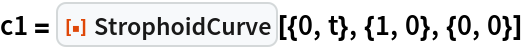Out=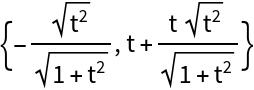The other branch of the strophoid:

 In:=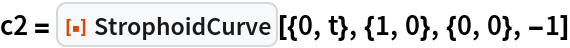Out=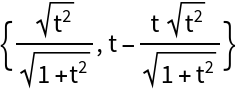Plot both branches together:

 In:=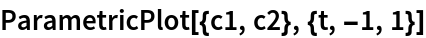Out=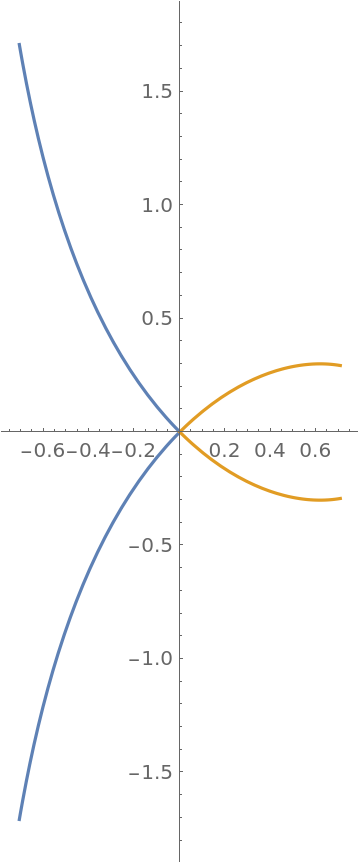Find the implicit Cartesian equation of the strophoid:

 In:=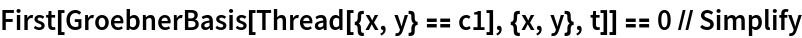Out=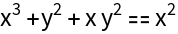The strophoid of a circle with pole at the circle's center, and fixed point at the circle's circumference:

 In:=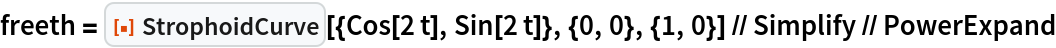Out=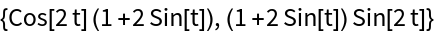Plot Freeth's nephroid:

 In:=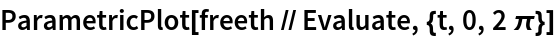Out=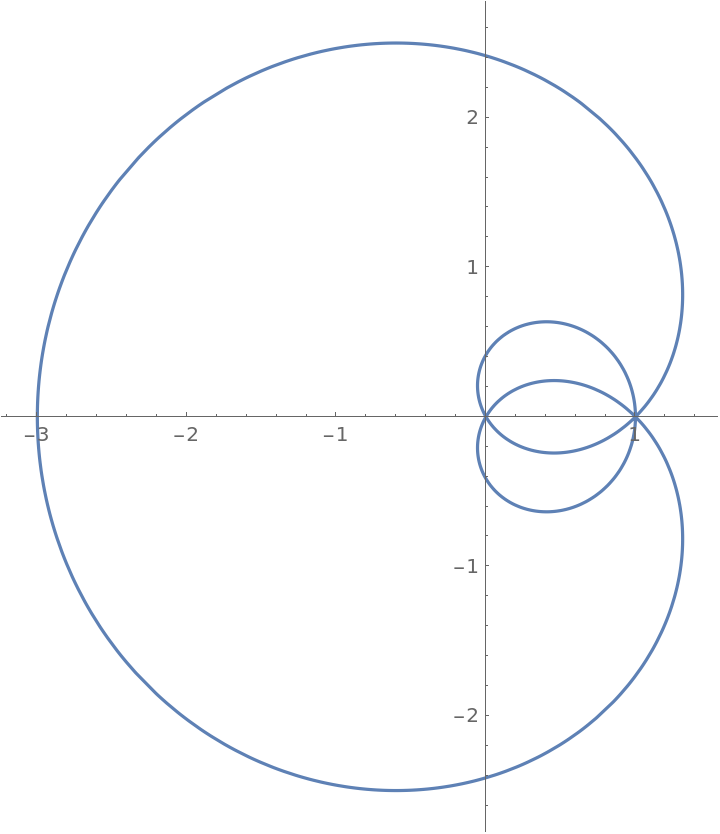The strophoid of a limaçon with pole at the origin, and fixed point at the node of the limaçon:

 In:=Out=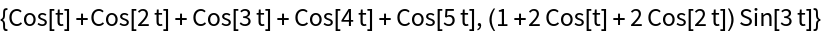Plot Freeth's supertrisectrix:

 In:=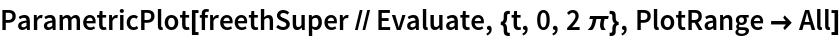Out=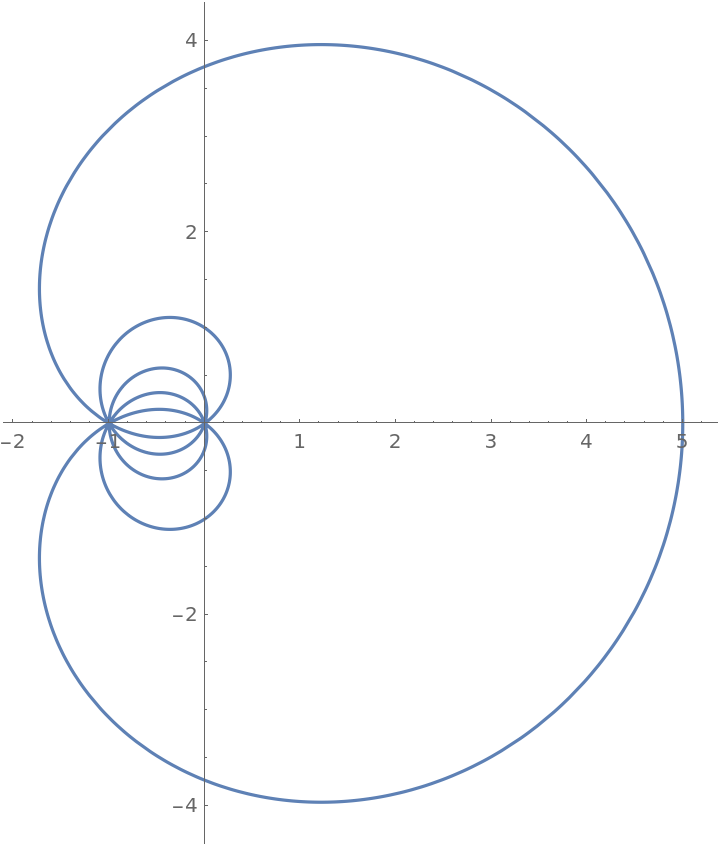## Version History

• 1.0.0 – 09 February 2021